Courses

# Properties Of Gasses And Gas Mixture - MCQ Test 2

## 30 Questions MCQ Test Mock Test Series - Mechanical Engineering (ME) for GATE 2020 | Properties Of Gasses And Gas Mixture - MCQ Test 2

Description
This mock test of Properties Of Gasses And Gas Mixture - MCQ Test 2 for Mechanical Engineering helps you for every Mechanical Engineering entrance exam. This contains 30 Multiple Choice Questions for Mechanical Engineering Properties Of Gasses And Gas Mixture - MCQ Test 2 (mcq) to study with solutions a complete question bank. The solved questions answers in this Properties Of Gasses And Gas Mixture - MCQ Test 2 quiz give you a good mix of easy questions and tough questions. Mechanical Engineering students definitely take this Properties Of Gasses And Gas Mixture - MCQ Test 2 exercise for a better result in the exam. You can find other Properties Of Gasses And Gas Mixture - MCQ Test 2 extra questions, long questions & short questions for Mechanical Engineering on EduRev as well by searching above.
QUESTION: 1

### A football was inflated to a gauge pressure of 1 bar when the ambient temperature was 15°C. When the game started next day, the air temperature at the stadium was 5°C. Assume that the volume of the football remains constant at 2500 cm3. The amount of heat lost by the air in the football and the gauge pressure of air in the football at the stadium respectively equal

Solution: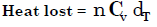QUESTION: 2

### A 100 W electric bulb was switched on in a 2.5 m × 3 m × 3 m size thermally insulated room having a temperature of 20°C. The room temperature at the end of 24 hours will be

Solution:

Heat produced by electric bulb in 24 hr.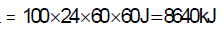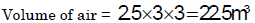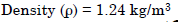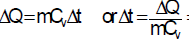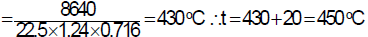QUESTION: 3

### 2 moles of oxygen are mixed adiabatically with another 2 moles of oxygen in a mixing chamber, so that the final total pressure and temperature of the mixture become same as those of the individual constituents at their initial states. The universal gas constant is given as R. The change in entropy due to mixing, per mole of oxygen, is given by

Solution:

Remember if we mix 2 mole of oxygen with another 2 mole of other gas the volume will be doubled for first and second constituents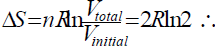Total Entropy change = 4Rln2 So, Entropy change per mole=Rln2. And it is due to diffusion of one gas into another.

QUESTION: 4

Assertion (A): A perfect gas is one that satisfies the equation of state and whose specific heats are constant.

Reason (R): The enthalpy and internal energy of a perfect gas are functions of temperature only.

Solution:

For perfect gas, both the assertion A and reason R are true. However R is not the explanation for A. A provides definition of perfect gas. R provides further relationship for enthalpy and internal energy but can't be reason for definition of perfect gas.

QUESTION: 5

Consider an ideal gas contained in vessel. If intermolecular interaction suddenly begins to act, which of the following happens?

Solution:
QUESTION: 6

Consider the following statements:

A real gas obeys perfect gas law at a very

1. High temperature

2. High-pressure

3. Low pressure

Which of the following statements is/are correct?

Solution:

In Perfect gas intermolecular attraction is zero. It will be only possible when intermolecular distance will be too high. High temperature or low pressure or both cause high intermolecular distance so choice 1 and 3.

QUESTION: 7

If a real gas obeys the Clausius equation of state p(v – b) = RT then,

Solution:
QUESTION: 8

Reduced pressure is

Solution:
QUESTION: 9

The internal energy of a gas obeying Van der Waal‟s equation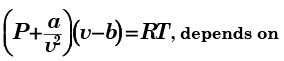Solution:

Joule‟s law states that for an Ideal gas internal energy is a function of temperature only. u = f(T). But this is not Ideal gas it is real gas.

QUESTION: 10

Which one of the following statements is correct?

Solution:
QUESTION: 11

The value of compressibility factor for an ideal gas may be:

1. less or more than one

2. equal to one

3. zero 4. less than zero

The correct value(s) is/are given by:

Solution:
QUESTION: 12

Assertion (A): An adiabatic process is always a constant entropy process.

Reason(R): In an adiabatic process there is no heat transfer.

Solution:
QUESTION: 13

In which of the following processes, the heat is fully converted into work?

Solution:
QUESTION: 14

An ideal gas undergoes an isothermal expansion from state R to state S in a turbine as shown in the diagram given below: The area of shaded region is 1000 Nm. What is the amount is turbine work done during the process?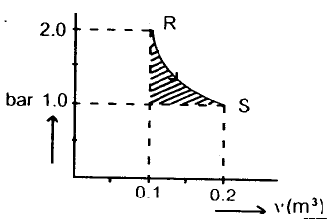Solution:

Turbine work = area under curve R–S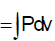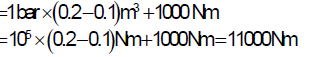QUESTION: 15

The slope of log P-log V graph for a gas for isothermal change is m1 and for adiabatic changes is m2. If the gas is diatomic gas, then

Solution:

PV = constant, C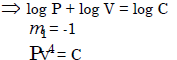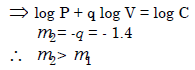QUESTION: 16

Air is being forced by the bicycle pump into a tyre against a pressure of 4-5 bars. A slow downward movement of the piston can be approximated as

Solution:
QUESTION: 17

The change in specific entropy of a system undergoing a reversible process is  given by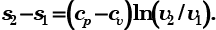This is valid for which one of the following?

Solution: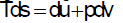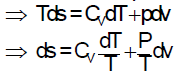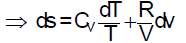Integrating the above expression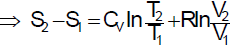For isothermal process undergone by ideal gas.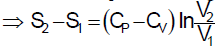QUESTION: 18

Change in enthalpy in a closed system is equal to the heat transferred, if the reversible process takes place at

Solution: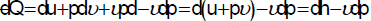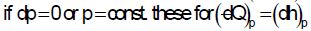QUESTION: 19

A saturated vapour is compressed to half its volume without changing its temperature. The result is that:

Solution:

By compressing a saturated vapour, its vapours condense and pressure remains unchanged. Remember it is not gas.

QUESTION: 20

Which one of the following thermodynamic processes approximates the steaming of food in a pressure cooker?

Solution:

Since the volume of pressure cooker is  constant hence it is an isochoric process.

QUESTION: 21

A system at a given state undergoes change through the following expansion processes to reach the same final volume

1. Isothermal

2. Isobaric

3. Adiabatic ( γ = 1.4)

4. Polytropic(n =1.3)

The correct ascending order of the work output in these four processes is

Solution:
QUESTION: 22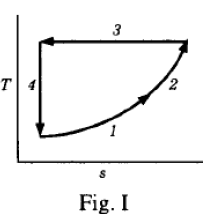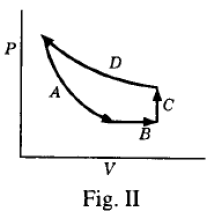Four processes of a thermodynamic cycle are shown above in Fig.I on the T-s plane in the sequence 1-2-3-4. The corresponding correct sequence of these processes in the p- V plane as shown above in Fig. II will be

Solution:
QUESTION: 23

A perfect gas at 27°C was heated until its volume was doubled using the following three different processes separately

1. Constant pressure process

2 Isothermal process

3. Isentropic process

Which one of the following is the correct sequence in the order of increasing value of the final temperature of the gas reached by using the above three different processes?

Solution: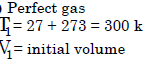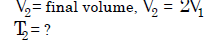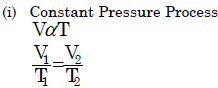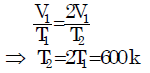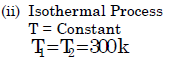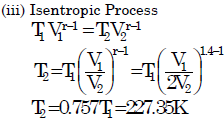QUESTION: 24

Assertion (A): For a perfect gas, hyperbolic expansion is an isothermal expansion.

Reason (R): For a perfect gas, PV/T = constant.

Solution:
QUESTION: 25

An ideal gas with initial volume, pressure and temperature of 0.1 m3, 1bar and 27°C respectively is compressed in a cylinder by a piston such that its final volume and pressure are 0.04 m3 and 5 bars respectively, then its final temperature will be:

Solution: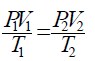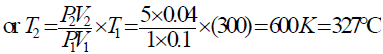QUESTION: 26

An Ideal gas with initial volume, pressure and temperature of 0.1m3, 1 bar and 27°C respectively is compressed in a cylinder by piston such that its final volume and pressure 0.04 m3 and 5 bar respectively, then its final temperature will be:

Solution:

Apply equation of states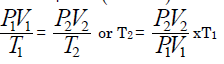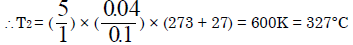QUESTION: 27

​If a gas obeys van der Waals' equation at the critical point, then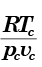is equal  to which one of the following

Solution: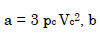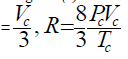QUESTION: 28

A higher value of Van der waal‟s constant for a gas indicates that the

Solution:
QUESTION: 29

Consider the following statements:

1. During a reversible non-flow process, for the same expansion ratio, work done by a gas diminishes as the value of n in pvn = C increases.

2. Adiabatic mixing process is a reversible process.

Which of the statements given above is/are correct?

Solution:

In adiabatic mixing there is always
increase in entropy so large amount of irreversibility is these.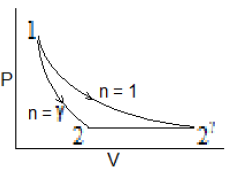QUESTION: 30

In a reversible isothermal expansion process, the fluid expands from 10 bar and 2 m3 to 2 bar and 10 m3. During the process the heat supplied is at the rate of 100 kW. What is the rate of work done during the process?

Solution:

For reversible isothermal expansion heat supplied is equal to work done during the process and equal to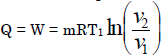∵ Temperature constant so no change in internal energy dQ = dU + dW; dU = 0 Therefore dQ = dW.